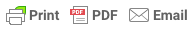Sorry, you do not have permission to ask a question, You must login to ask question. Become VIP Member

Do you need to remove the ads? Become VIP Member

Modulus of elasticity of concrete is defined as the ratio of stress applied on the concrete to the respective strain caused. The accurate value of modulus of elasticity of concrete can be determined by conducting a laboratory test called compression test on a cylindrical concrete specimen.

In the test, the deformation of the specimen with respect to different load variation is analyzed. These observations produce Stress-Strain graph (load-deflection graph) from which the modulus of elasticity of concrete is determined. The slope of a line that is drawn in the stress-strain curve from a stress value of zero to the compressive stress value of 0.45f’c(working stress) gives the modulus of elasticity of concrete.

The laboratory test to determine the modulus of elasticity of concrete is explained below.

## Procedure

The test procedure involves two stages. Initially, the compressometer is set-up, followed by the application of load and testing.

### Setting Up Compressometer

A compressometer is a device used in the compression test of the concrete cylinder to determine its strain and deformation characteristics. The set up involves the following procedures.

1. The compressometer consists of two frames(top and bottom), as shown in figure-1. The frames are initially assembled by the help of spacers. The spacers are held in position during the assembling.
2. The pivot rod is kept on the screws which are then locked in position. The tightening screws of the top and bottom frames are kept in loose condition.
3. Once the compressometer is arranged, it is placed on the concrete specimen kept on a level surface. The compressometer is centrally placed on the specimen.
4. Once the position is set, the screws are tightened and the compressometer is held on the specimen.
5. Once the set up is done, the spacers can be unscrewed and removed.

### Testing the Specimen

The test procedure involves the following steps:

1. The specimen with the compressometer set up is placed over the compression testing machine platform. It is centered properly.
2. The load application is performed continuously at a rate of 140 kg/cm²/minute without any obstruction.
3. The load application is continued until a stress value equal to (c+5)kg/cm² is attained. Here c is the 1/3rd of average compressive strength of the cube ( The strength value of cube calculated to the nearest of 5kg /cm²) which is a load of 12.4T.
4. Once this stress value is reached, it is maintained for a period of 60 seconds and then reduced to the stress of 1.5 kg/cm² which is a load value of 0.3T.
5. Again, the load is further increased until the stress of (c+1.5) kg/cm² is reached which is a load of 11.8T. At this point, compressometer reading is recorded.
7. Repeat the test by applying the load for the third time and record the compressometer’s readings at an interval of 1T i.e 1.8T,10.8T,9.8T,8.8T,7.8T,…………,1.8T,0.3T is determined.

From the observations, the load deflection graph is plotted for the loading conditions. Tangents are drawn at the initial portion of the graph and at the point of value equal to the working stress of the concrete mix. A line is drawn joining both points.& Ad Free!

GET VIP MEMBERSHIP NOW!

Read More: Stress-Strain Curve of Concrete

## Calculation and Results

### Calculation

Slope of Initial Tangent gives:

Initial tangent modulus = stress/strain

Slope of tangent at working stress gives:

Tangent modulus at working stress= stress/strain

Slope of Line joining initial tangent point and point of working stress gives:

Secant modulus = stress/strain

### Test Report

The following information shall be included in the report.

• Identification mark
• Date of test
• Age of specimen
• Shape and nominal dimensions of the specimen

### Result

Initial tangent modulus of given concrete = ………………N / mm²

Tangent modulus at working stress =……………….N / mm²

Secant modulus (Modulus of elasticity of given concrete) = ………….. N / mm²

## Precautions

1. Reading must be taken continuously without any delay
2. If the strain readings differ by more than 5% for the different trials, then the test must be repeated.

EDITOR

## Major Problems in Concrete Pumping Works: How to Avoid Them?

### 1 Comment

1.thanks

• Share